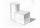# Diagonal + expression of a variable from formula - math problems

1. Faces diagonalsIf the diagonals of a cuboid are x, y, and z (wall diagonals or three faces) respectively than find the volume of a cuboid. Solve for x=1.2, y=1.7, z=1.45
2. Square diagonalCalculate length of the square diagonal if the perimeter is 476 cm.
3. IS trapezoidCalculate the length of diagonal u and height v of isosceles trapezoid ABCD, whose bases have lengths a = |AB| = 37 cm, c = |CD| = 29 cm and legs b = d = |BC| = |AD| = 28 cm.
4. Rhombus 2Calculate the area of rhombus which has a height v=48 mm and shorter diagonal u = 60 mm long.
5. Square diagonalCalculate the length of diagonal of the square with side a = 23 cm.
6. Rectangle diagonalsIt is given rectangle with area 24 cm2 a circumference 20 cm. The length of one side is 2 cm larger than length of second side. Calculate the length of the diagonal. Length and width are yet expressed in natural numbers.
7. RectangleThe length of the rectangle are in the ratio 5:12 and the circumference is 238 cm. Calculate the length of the diagonal and area of rectangle.
8. CarpetThe room is 10 x 5 meters. You have the role of carpet width of 1 meter. Make rectangular cut of roll that piece of carpet will be longest possible and it fit into the room. How long is a piece of carpet? Note .: carpet will not be parallel with the diag
9. Prism - boxThe base of prism is a rectangle with a side of 7.5 cm and 12.5 cm diagonal. The volume of the prism is V = 0.9 dm3. Calculate the surface of the prism.
10. Rhombus diagonalArea of rhombus is 224. One diagonal measures 33, find length of other diagonal.
11. Square pyramidCalculate the volume of the pyramid with the side 5cm long and with a square base, side-base has angle of 60 degrees.
12. Diagonal 20Diagonal pathway for the rectangular town plaza whose length is 20 m longer than the width. if the pathway is 20 m shorter than twice the width. How long should the pathway be?
13. Trapezoid thirdsThe ABCD trapezoid with the parallel sides of the AB and the CD and the E point of the AB side if the segment DE divides the trapezoid into two parts with the same area. Find the length of the AE line segment.
14. The fieldThe player crossed the field diagonally and walked the length of 250 m. Calculate the length of the field, circumference if one side of field 25 meters.
15. RhombusOne angle of a rhombus is 136° and the shorter diagonal is 8 cm long. Find the length of the longer diagonal and the side of the rhombus.
16. Diamond diagonalsCalculate the diamond's diagonal lengths if its content is 156 cm2 and the side length is 13 cm.
17. Body diagonalCuboid with base 7cm x 3,9cm and body diagonal 9cm long. Find the height of the cuboid and the length of the diagonal of the base,
18. Rhombus and diagonalsThe a rhombus area is 150 cm2 and the ratio of the diagonals is 3:4. Calculate the length of its height.
19. Circle and rectangleA rectangle with sides of 11.7 cm and 175 mm is described by circle. What is its length? Calculate the content area of the circle described by this circle.
20. Surface area of cylinderDetermine the lateral surface of the rotary cylinder which is circumscribed cube with edge length 5 cm.

Do you have an interesting mathematical word problem that you can't solve it? Enter it, and we can try to solve it.

To this e-mail address, we will reply solution; solved examples are also published here. Please enter the e-mail correctly and check whether you don't have a full mailbox.

Please do not submit problems from current active competitions such as Mathematical Olympiad, correspondence seminars etc...